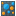Versions

You are viewing the RapidMiner Studio documentation for version 8.1 - Check here for latest versionCovariance Matrix (RapidMiner Studio Core)

Synopsis

This operator calculates the covariance between all attributes of the input ExampleSet and returns a covariance matrix giving a measure of how much two attributes change together.

Description

Covariance is a measure of how much two attributes change together. If the greater values of one attribute mainly correspond with the greater values of the other attribute, and the same holds for the smaller values, i.e. the attributes tend to show similar behavior, the covariance is a positive number. In the opposite case, when the greater values of one attribute mainly correspond to the smaller values of the other, i.e. the attributes tend to show opposite behavior, the covariance is negative. The sign of the covariance therefore shows the tendency in the linear relationship between the variables. For two attributes x and y having means E{x} and E{y}, the covariance is defined as:

Cov(x,y) = E{[ x - E(x) ][ y - E(y) ]}

The covariance calculation begins with pairs of x and y, takes their differences from their mean values and multiplies these differences together. For instance, if for x1 and y1 this product is positive, for that pair of data points the values of x and y have varied together in the same direction from their means. If the product is negative, they have varied in opposite directions. The larger the magnitude of the product, the stronger the strength of the relationship. The covariance is defined as the mean value of this product, calculated using each pair of data points x(i) and y(i). If the covariance is zero, then the cases in which the product was positive were offset by those in which it was negative, and there is no linear relationship between the two attributes.

The value of the covariance is interpreted as follows:

• Positive covariance: indicates that higher than average values of one attribute tend to be paired with higher than average values of the other attribute.
• Negative covariance: indicates that higher than average values of one attribute tend to be paired with lower than average values of the other attribute.
• Zero covariance: if the two attributes are independent, the covariance will be zero. However, a covariance of zero does not necessarily mean that the variables are independent. A nonlinear relationship can exist that still would result in a covariance value of zero.

Because the number representing covariance depends on the units of the data, it is difficult to compare covariances among data sets having different scales. A value that might represent a strong linear relationship for one data set might represent a very weak one in another.

Input

•example set (Data Table)

This input port expects an ExampleSet. It is the output of the Retrieve operator in the attached Example Process. The output of other operators can also be used as input.

Output

•example set (Data Table)

The ExampleSet that was given as input is passed without changing to the output through this port. This is usually used to reuse the same ExampleSet in further operators or to view the ExampleSet in the Results Workspace.

•covariance (Numerical Matrix)

The covariances of all attributes of the input ExampleSet are calculated and the resultant covariance matrix is returned from this port.

Tutorial Processes

Covariance matrix of the Polynomial data set

The 'Polynomial' data set is loaded using the Retrieve operator. A breakpoint is inserted here so that you can view the ExampleSet. As you can see that the ExampleSet has 5 real attributes. The Covariance Matrix operator is applied on this ExampleSet. The resultant covariance matrix can be viewed in the Results Workspace.